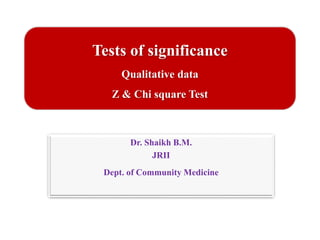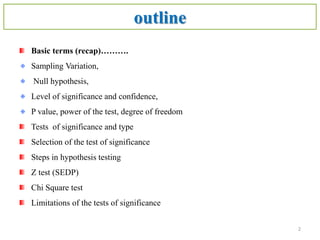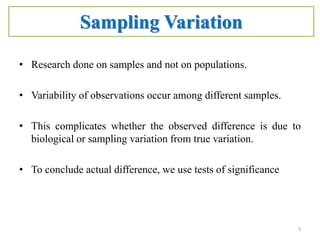Successfully reported this slideshow.

# Test of-significance : Z test , Chi square test

87

Share×
1 of 35
1 of 35

# Test of-significance : Z test , Chi square test

87

Share

Test of significance and qualitative tests

Test of significance and qualitative tests

## More Related Content

### Related Books

Free with a 14 day trial from Scribd

See all

### Related Audiobooks

Free with a 14 day trial from Scribd

See all

### Test of-significance : Z test , Chi square test

1. 1. Tests of significance Qualitative data Z & Chi square Test Dr. Shaikh B.M. JRII Dept. of Community Medicine
2. 2. outline Basic terms (recap)………. Sampling Variation, Null hypothesis, Level of significance and confidence, P value, power of the test, degree of freedom Tests of significance and type Selection of the test of significance Steps in hypothesis testing Z test (SEDP) Chi Square test Limitations of the tests of significance 2
3. 3. Sampling Variation • Research done on samples and not on populations. • Variability of observations occur among different samples. • This complicates whether the observed difference is due to biological or sampling variation from true variation. • To conclude actual difference, we use tests of significance 3
4. 4. Test of significance • The test which is done for testing the research hypothesis against the null hypothesis. Why it is done?  To assist administrations and clinicians in making decision.  The difference is real ? or  Has it happened by chance ? 4
5. 5. Null Hypothesis (HO) • 1st step in testing any hypothesis. • Set up such that it conveys a meaning that there exists no difference between the different samples. • Eg: Null Hypothesis – The mean pulse rate among the two groups are same (or) there is no significant difference between their pulse rates. 5
6. 6. • By using various tests of significance we either: –Reject the Null Hypothesis (or) –Accept the Null Hypothesis • Rejecting null hypothesis → difference is significant. • Accepting null hypothesis → difference is not significant. 6
7. 7. Level of significance and confidence • Significance means the percentage risk to reject a null hypothesis when it is true and it is denoted by 𝛼.Generally takenas1%,5%,10% • (1 − 𝛼) is the confidencelevelin which the null hypothesis will exist when it is true. 7
8. 8. Level of Significance – “P” Value • p-value is a function of the observed sample results (a statistic) that is used for testing a statistical hypothesis. • It is the probability of null hypothesis being true. It can accept or reject the null hypothesis based on P value. • Practically, P < 0.05 (5%) is considered significant. 8
9. 9. • P = 0.05 implies, – We may go wrong 5 out of 100 times by rejecting null hypothesis. – Or, We can attribute significance with 95% confidence. 9
10. 10. 5% Significance level & 95% confidence level Acceptance and Rejection regions 𝑅𝑒𝑗𝑒𝑐𝑡𝑖𝑜𝑛 𝑟𝑒𝑔𝑖𝑜𝑛 /𝑠𝑖𝑔𝑛𝑖𝑓𝑖𝑐𝑎𝑛𝑐𝑒 𝑙𝑒𝑣𝑒𝑙 (𝛼 = 0.025 𝑜𝑟 2.5%) 𝑅𝑒𝑗𝑒𝑐𝑡𝑖𝑜𝑛 𝑟𝑒𝑔𝑖𝑜𝑛 /𝑠𝑖𝑔𝑛𝑖𝑓𝑖𝑐𝑎𝑛𝑐𝑒 𝑙𝑒𝑣𝑒𝑙 (𝛼 = 0.025 𝑜𝑟 2.5%) 𝑇𝑜𝑡𝑎𝑙 𝐴𝑐𝑐𝑒𝑝𝑡𝑎𝑛𝑐𝑒 𝑟𝑒𝑔𝑖𝑜𝑛 𝑜𝑟 𝑐𝑜𝑛𝑓𝑖𝑑𝑒𝑛𝑐𝑒 𝑙𝑒𝑣𝑒𝑙 (1 − 𝛼) = 95% 10 10
11. 11. Power of the test & Degree of freedom Power of the test : Type II error is β and 1−β is called power of the test. Probability of rejecting False H0, i. e. taking correct decision Degree of freedom: • Number of independent observations used in statistics (d. f.) 11
12. 12. Various tests of significance 1. Parametric – Data is or assumed to be normal distributed. 2. Non parametric – Data is not normal distributed.  Parametric tests : For Qualitative data:- 1. Z test 2. 2. Chi-square test or X2 For Quantitative data:- 1. Unpaired ‘t’ test 2. Paired ‘t’ test 3. ANOVA 12
13. 13. Selection of the test • Base 1. Type of data 2. Size of sample 3. Number of samples 13
14. 14. Selection of the test 14 Qualitative Data n > 30 Large Sample 1, 2 samples Z test n </> 30 Small / large Sample More than 2 samples Chi square test Quantitative Data n </> 30 Small / large Sample 1 samples One sample t-test 2 samples Unpaired t- test / Paired t- test More than 2 samples ANOVA
15. 15. Steps in Testing a Hypothesis • General procedure in testing a hypothesis 1. Set up a null hypothesis (HO). 2. Define alternative hypothesis (HA). 3. Calculate the test statistic (Z, X2, t etc.). 4. Find out the corresponding probability level (P Value) for the calculated test statistic from relevant tables. 5. Accept or reject the Null hypothesis depending on P value. P> 0.05 HO accepted P < 0.05 HO rejected 15
16. 16. Z test [Standard error of difference between proportions (SEp1-p2)] • For comparing qualitative data between 2 groups. • Used for large samples only. (> 30 in each group). • Standard error of difference between proportions (SEp1-p2) is calculated by using the formula 16
17. 17. • And then the test statistic ‘Z Score’ value is calculated by using the formula: • If Z > 1.96 P < 0.05 SIGNIFICANT - H0 rejected • If Z < 1.96 P > 0.05 NOT SIGNIFICANT- H0 accepted • Finding p value from Z table • Go to the row that represents the ones digit and the first digit after the decimal point (the tenths digit) of your z-value. • Go to the column that represents the second digit after the decimal point (the hundredths digit) of your z-value. • Intersect the row and column from Steps 1 and 2. 1.0000 - 0.9772 =0.0228 < 0.05 17
18. 18. Example • Consider a hypothetical study where cure rate of Typhoid fever after treatment with Ciprofloxacin and Ceftriaxone were recorded to be 90% and 80% among 100 patients treated with each of the drug. • How can we determine whether cure rate of Ciprofloxacin is better than Ceftriaxone? 18
19. 19. Solution Step – 1: Set up a null hypothesis – H0: – “There is no significant difference between cure rates of Ciprofloxacin and Ceftriaxone.” Step – 2: Define alternative hypothesis – Ha: – “Ciprofloxacin is 1.125 times better in curing typhoid fever than Ceftriaxone.” Step – 3: Calculate the test statistic – ‘Z Score’ 19
20. 20. Step – 3: Calculating Z score – Here, P1 = 90, P2 = 80 – SEP1-P2 will be given by the formula: So, Z = 10/5 = 2 20
21. 21. Step – 4: Find out the corresponding P Value – Since Z = 2 i.e., > 1.96, hence, P < 0.05 Step – 5: Accept or reject the Null hypothesis – Since P < 0.05, So we reject the null hypothesis(H0) There is no significant difference between cure rates of Ciprofloxacin and Ceftriaxone – And we accept the alternate hypothesis (Ha) that, Ciprofloxacin is 1.125 times better in curing typhoid fever than Ceftriaxone 21
22. 22. Chi square (X2) test • This test is also for testing qualitative data. • Its advantage over Z test is: – Can be applied for smaller samples as well as for large samples. • Prerequisites for Chi square (X2) test to be applied: – The sample must be a random sample – None of the observed values must be zero. – Adequate cell size 22
23. 23. Steps in Calculating (X2) value 1. Make a contingency table mentioning the frequencies in all cells. 2. Determine the expected value (E) in each cell. 3. Calculate the difference between observed and expected values in each cell (O-E). 23
24. 24. 4. Calculate X2 value for each cell 5. Sum up X2 value of each cell to get X2 value of the table. 6. Find out p value from x2 table 7. If p > 0.05 , difference is not significant, null hypothesis accepted; If p < 0.05 , difference is significant , null hypothesis rejected. 24
25. 25. Example • Consider a study done in a hospital where cases of breast cancer were compared against controls from normal population against with a family history of Ca Breast. • 100 in each group were studied for presence of family history. • 25 of cases and 15 among controls had a positive family history. • Comment on the significance of family history in breast cancer. 25
26. 26. Solution • From the numbers, it suggests that family history is 1.66 (25/15) times more common in Ca breast. • So is it a risk factor in population? • We need to test for the significance of this difference. • We shall apply X2 test. 26
27. 27. Solution Step – 1: Set up a null hypothesis – H0: “There is no significant difference between incidence of family history among cases and controls.” Step – 2: Define alternative hypothesis – Ha: “Family history is 1.66 times more common in Ca breast” 27
28. 28. Step – 3: Calculating X2 1. Make a contingency table mentioning the frequencies in all cells 28
29. 29. Step – 3: Cont.. 2. Determine the expected value (E) for each cell. 29
30. 30. O – observed values , E – expected value 30
31. 31. Step – 4: Determine degree of freedom. • DoF is determined by the formula: DoF = (r-1) x (c-1) where r and c are the number of rows and columns respectively • Here, r = c = 2. • Hence, DoF = (2-1) x (2-1) = 1 31
32. 32. Step – 5: Find out the corresponding P Value – P values can be derived by using the X2 distribution tables 32
33. 33. Step – 6: Accept or reject the Null hypothesis • In given scenario, X2 = 3.125 • This is less than 3.84 (for P = 0.05 at dof =1) • Hence Null hypothesis is Accepted, i.e., “There is no significant difference between incidence of family history among cases and controls” 33
34. 34. Limitations of tests of significance  Testing of hypothesis is not decision making itself; but it helps for decision making  Test does not explain the reasons as why the difference exists, tests do not tell about the reason causing the difference.  Tests are based on the probabilities and as such can not be expressed with full certainty.  Statistical inferences based on the significance tests can not be said to be entirely correct evidences concerning the truth of the hypothesis. 34
35. 35. THANK YOU 35